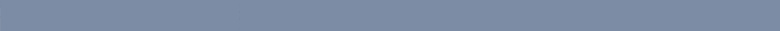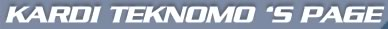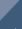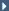HomeNumerical Excel TutorialMicroscopic Pedestrian SimulationKardi Teknomo's TutorialMicro-PedSim Free DownloadPersonal Development Handbook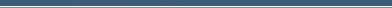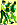Research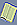Publications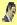Tutorials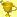Resume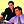Personal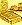Resources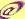Contact

Mathematics as practical problem solverMathematic is definitely a great weapon to solve many problems.

I am talking about general mathematics here which include statistics, logic and computer programming.

Once we can translate our problem into mathematics language, the whole mathematical principles and theorems are ready to attack the problem rationally.

Of course we need to translate back to real world after we get the solution from the mathematics language.

The weakness of mathematics is in the translation (human have not acquire this systematic technology until now). If we translate it wrong, the result will be wrong even though it is mathematically correct.